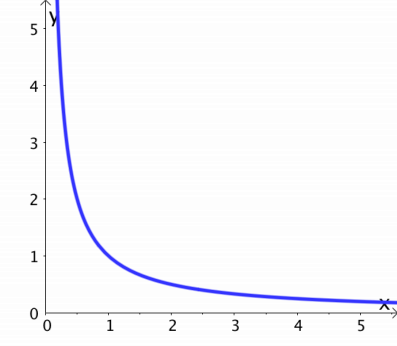Math Topics

# Inversely Proportional Functions

Two variables $x$ and $y$ are inversely proportional if their product is constant. That means you always get the same answer when you multiply $x$ and $y$ together.

Theory

### InverseProportionality

Two variables $x$ and $y$ are inversely proportional if

 $y=\frac{k}{x}$

where $k$ is a constant.

Example 1

Saying that $y=\frac{k}{x}$ is the same as saying that $x\cdot y=k$: $\begin{array}{llllllll}\hfill x\cdot y& =k,\phantom{\rule{2em}{0ex}}& \hfill & \phantom{\rule{1em}{0ex}}x\ne 0\phantom{\rule{2em}{0ex}}& \hfill & \phantom{\rule{2em}{0ex}}& \hfill & \phantom{\rule{2em}{0ex}}\\ \hfill \frac{x\cdot y}{x}& =\frac{k}{x},\phantom{\rule{2em}{0ex}}& \hfill & \phantom{\rule{1em}{0ex}}x\ne 0\phantom{\rule{2em}{0ex}}& \hfill & \phantom{\rule{2em}{0ex}}& \hfill & \phantom{\rule{2em}{0ex}}\\ \hfill y& =\frac{k}{x},\phantom{\rule{2em}{0ex}}& \hfill & \phantom{\rule{1em}{0ex}}x\ne 0\phantom{\rule{2em}{0ex}}& \hfill & \phantom{\rule{2em}{0ex}}& \hfill & \phantom{\rule{2em}{0ex}}\end{array}$

Example 2

This graph shows $y=\frac{1}{x}$, that is, $k=1$. The graph is inversely proportional, so for all points on the graph, if you multiply the $x$-coordinate by the $y$-coordinate, the answer is $k=1$.Example 3

Is the graph $y=\frac{2}{3x}$ inversely proportional?

You can find that out by doing a few conversions: $\begin{array}{llll}\hfill y& =\frac{2}{3x}\phantom{\rule{2em}{0ex}}& \hfill & \phantom{\rule{2em}{0ex}}\\ \hfill & =\frac{2\cdot 1}{3\cdot x}\phantom{\rule{2em}{0ex}}& \hfill & \phantom{\rule{2em}{0ex}}\\ \hfill & =\frac{2}{3}\cdot \frac{1}{x}\phantom{\rule{2em}{0ex}}& \hfill & \phantom{\rule{2em}{0ex}}\\ \hfill & \approx 0.67\cdot \frac{1}{x}\phantom{\rule{2em}{0ex}}& \hfill & \phantom{\rule{2em}{0ex}}\\ \hfill & \approx \frac{0.67}{x}\phantom{\rule{2em}{0ex}}& \hfill & \phantom{\rule{2em}{0ex}}\end{array}$

You’ve found that $k=\frac{2}{3}\approx 0.67$, so the graph is inversely proportional.

Example 4

You have been given the following points:

 $x$-values 1 2 3 4 5 $y$-values 20 10 7 5 4

Do the points follow an inversely proportional function?

From the theory, you know that if multiply the $x$-value by the $y$-value and the answer is the same for all points, then the points follow an inversely proportional function. You check the points from the table: $\begin{array}{llll}\hfill 1\cdot 20& =20\phantom{\rule{2em}{0ex}}& \hfill & \phantom{\rule{2em}{0ex}}\\ \hfill 2\cdot 10& =20\phantom{\rule{2em}{0ex}}& \hfill & \phantom{\rule{2em}{0ex}}\\ \hfill 3\cdot 7& =21\phantom{\rule{2em}{0ex}}& \hfill & \phantom{\rule{2em}{0ex}}\\ \hfill 4\cdot 5& =20\phantom{\rule{2em}{0ex}}& \hfill & \phantom{\rule{2em}{0ex}}\\ \hfill 5\cdot 4& =20\phantom{\rule{2em}{0ex}}& \hfill & \phantom{\rule{2em}{0ex}}\end{array}$

Because one of the answers is not the same as the others, they do not follow an inversely proportional function.